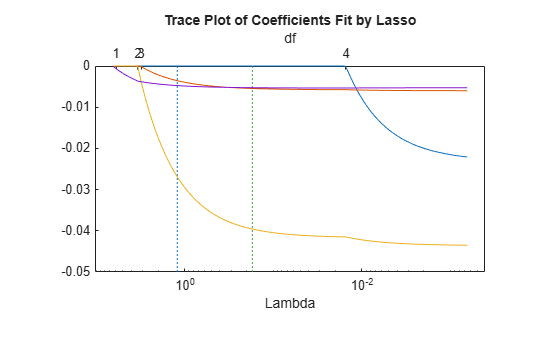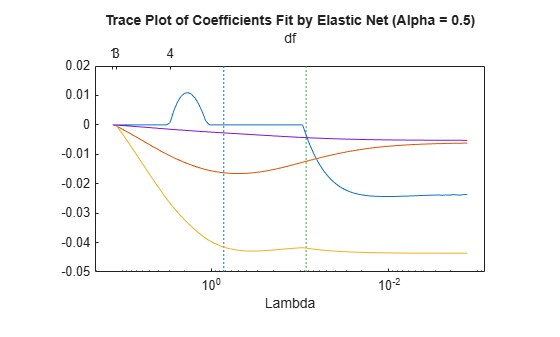Lasso and Elastic Net with Cross Validation

This example shows how to predict the mileage (MPG) of a car based on its weight, displacement, horsepower, and acceleration, using the lasso and elastic net methods.

Extract the continuous (noncategorical) predictors (lasso does not handle categorical predictors).

X = [Acceleration Displacement Horsepower Weight];

Perform a lasso fit with 10-fold cross validation.

[b,fitinfo] = lasso(X,MPG,'CV',10);

Plot the result.

lassoPlot(b,fitinfo,'PlotType','Lambda','XScale','log');Calculate the correlation of the predictors. Eliminate NaNs first.

nonan = ~any(isnan([X MPG]),2);
Xnonan = X(nonan,:);
MPGnonan = MPG(nonan,:);
corr(Xnonan)
ans = 4×4

1.0000   -0.5438   -0.6892   -0.4168
-0.5438    1.0000    0.8973    0.9330
-0.6892    0.8973    1.0000    0.8645
-0.4168    0.9330    0.8645    1.0000

Because some predictors are highly correlated, perform elastic net fitting. Use Alpha = 0.5.

[ba,fitinfoa] = lasso(X,MPG,'CV',10,'Alpha',.5);

Plot the result. Name each predictor so you can tell which curve is which.

pnames = {'Acceleration','Displacement','Horsepower','Weight'};
lassoPlot(ba,fitinfoa,'PlotType','Lambda','XScale','log',...
'PredictorNames',pnames);When you activate the data cursor and click the plot, you see the name of the predictor, the coefficient, the value of Lambda, and the index of that point, meaning the column in b associated with that fit.

Here, the elastic net and lasso results are not very similar. Also, the elastic net plot reflects a notable qualitative property of the elastic net technique. The elastic net retains three nonzero coefficients as Lambda increases (toward the left of the plot), and these three coefficients reach 0 at about the same Lambda value. In contrast, the lasso plot shows two of the three coefficients becoming 0 at the same value of Lambda, while another coefficient remains nonzero for higher values of Lambda.

This behavior exemplifies a general pattern. In general, elastic net tends to retain or drop groups of highly correlated predictors as Lambda increases. In contrast, lasso tends to drop smaller groups, or even individual predictors.